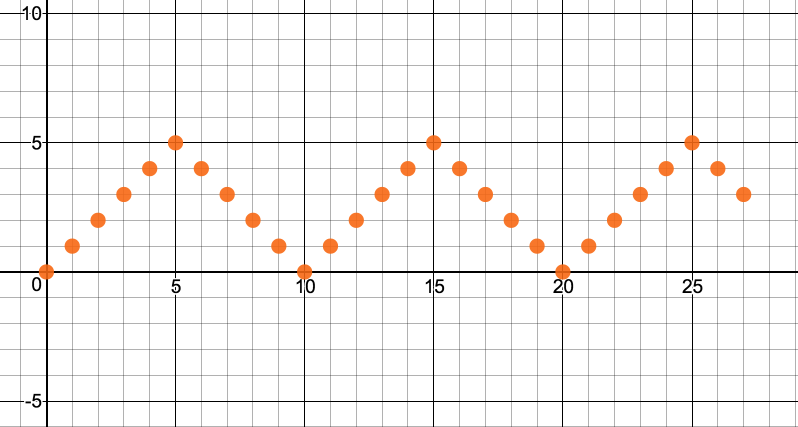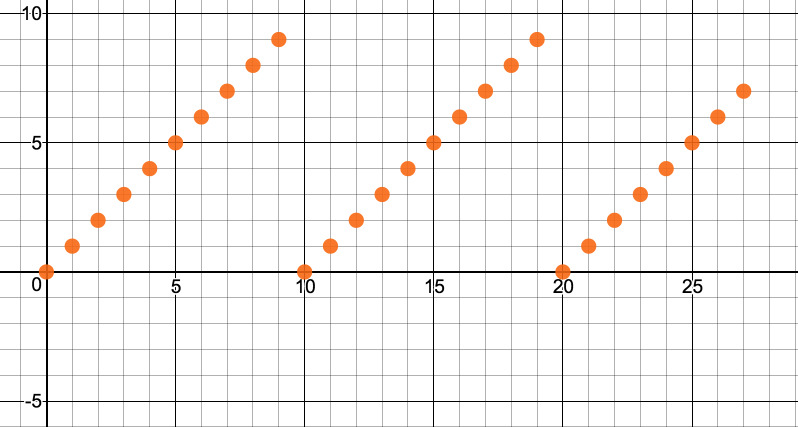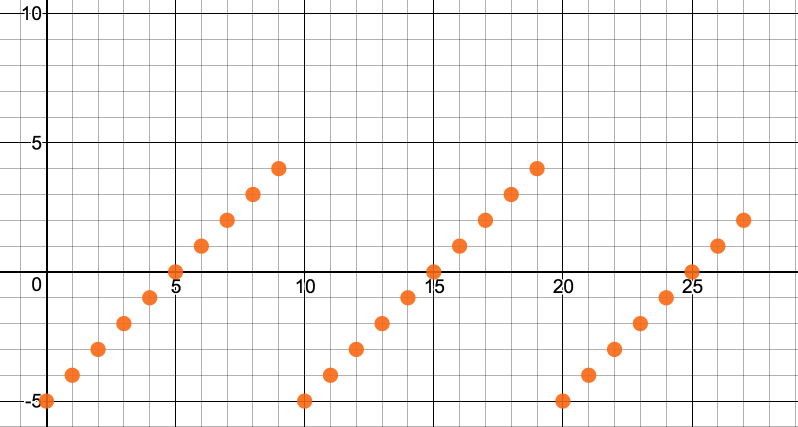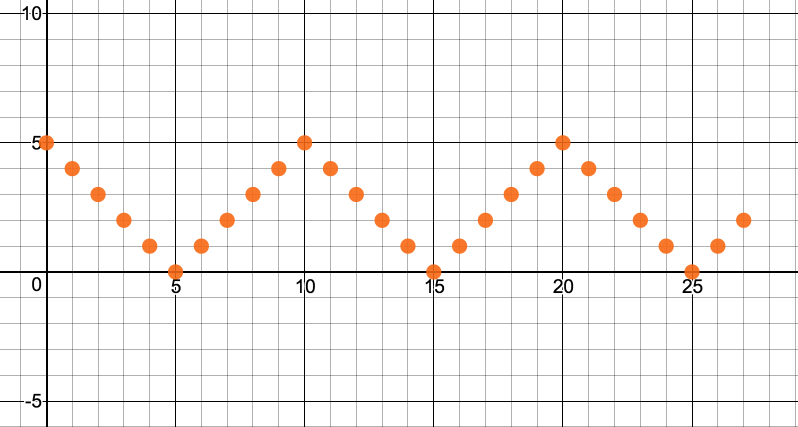# teaching machines

## Integer Triangle Wave

I encounter many of the same problems over and over again. But sometimes enough time elapses between one encounter with a problem and the next that I’ve forgotten how to solve it. I start to doubt my qualifications as a teacher. “That guy can’t even learn. How could we trust him to teach?” I think the problem is that I just don’t reflect on my thinking enough for the solution to stick.

One of those problems is generating a triangular wave of integers. I ran into it most recently in the Advent of Code, day 13. Here’s my attempt at thinking it through a little more carefully.

I want to generate a pattern that looks like this:Such an oscillator is nice for positioning an object as it bounces from one side of the screen to the other. It also models an elevator operating in Sabbath mode. (I stayed at a hotel in Haifa with one of these. From Friday evening to Saturday evening, it stops at every floor without the rider needing to press any buttons and thereby violating Sabbath rules.)

In this example, the period is 10.

Certainly, one could use an if statement to arbitrate between the rising and falling, but that feels wrong. I just want to use math. Well, math over the integers. Some people don’t think that’s math.

My first instinct is to use

$$y = \textrm{mod}(x, \textrm{period})$$

where x represents the passage of discrete time. This produces a nice sawtooth pattern:But I don’t want sawtooth. I know that to get the mountain peak pattern, I’ll need to use an absolute value somewhere. For absolute value to introduce discontinuities, some points will need to be below the y-axis. So let’s shift half of them below:

$$\textrm{mod}(x, \textrm{period}) – \frac{\textrm{period}}{2}$$Now absolute value should shake things up a bit! Let’s apply it:

$$\left|\textrm{mod}(x, \textrm{period}) – \frac{\textrm{period}}{2}\right|$$That’s just about it. Usually I want the wave to start at 0. We could accomplish that with a phase shift, or we could simply invert the whole thing.

$$\frac{\textrm{period}}{2} – \left|\textrm{mod}(x, \textrm{period}) – \frac{\textrm{period}}{2}\right|$$We got it!

The graph I used to think this through is on Desmos.